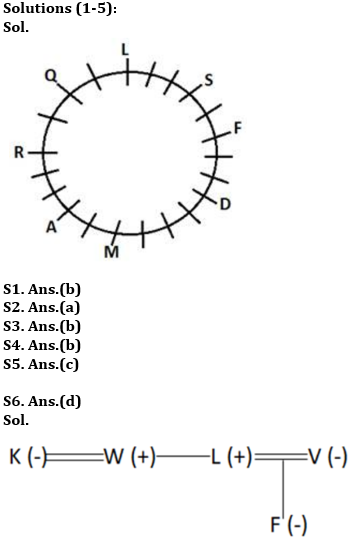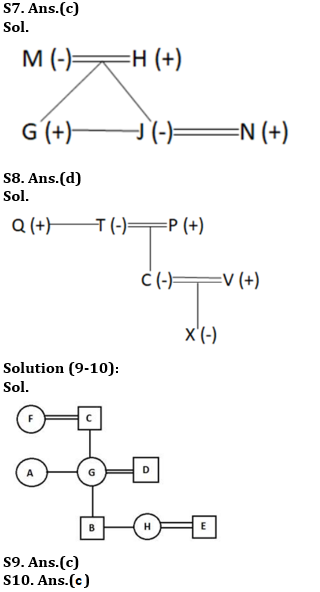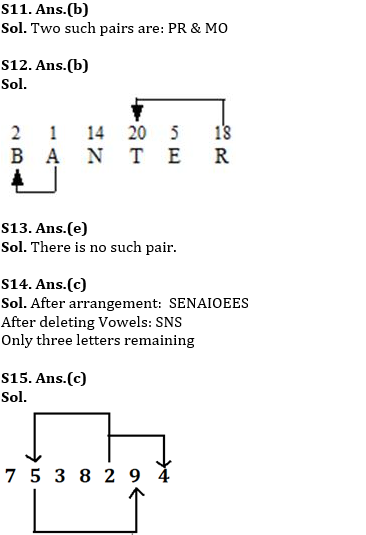Latest Banking jobs   »

# Reasoning Ability Quiz For RBI Assistant/ ESIC UDC Mains 2022- 13th April

Directions (1-5): Study the following information carefully and answer the given questions.
A certain number of people sit around a circular table and face outside the table. S sits third to the right of L. One person sits between S and F. The number of people sits between L and S when counted from the left of S is the same as the number of people sit between A and R when counted from the right of A. Two people sit between F and D who doesn’t sit adjacent to S. The number of people sits between R and L when counted from the right of R is one more than the number of people sit between F and D when counted from the left of D. Three people sit between D and M who doesn’t sit between F and S. A sits second to the right of M. More than two people sit between A and D, when counted either right or left of D. Number of people seated between Q and L equals the number of people seated between R and Q.

Q1. Who amongst the following sits exactly between A and S, when counted clockwise from A?
(a) D
(b) Q
(c) R
(d) F
(e) None of these

Q2. How many people sit around the circular table?
(a) Twenty one
(b) Nineteen
(c) Twenty
(d) Eighteen
(e) None of these

Q3. What is the position of R with respect to D?
(a) Ninth to the left
(b) Ninth to the right
(c) Eighth to the left
(d) Eighth to the right
(e) None of these

Q4. Who amongst the following sits ninth to the right of M?
(a) F
(b) L
(c) Q
(d) S
(e) None of these

Q5. If S is related to F and M is related to A then in the same manner Q is related to whom?
(a) R
(b) S
(c) L
(d) M
(e) None of these

Directions (6-8): Study the data carefully and answer the questions accordingly.
A @ B means A is the father of B
A # B means A is the daughter of B
A % B means A is the brother of B
A * B means A is the wife of B

Q6. If K * W % L @ F # V, then how V is related to W?
(a) Niece
(b) Aunt
(c) Sister
(d) Sister-in-law
(e) None of these

Q7. Which of the following shows than N is the son-in-law of H?
(a) M * H % G % J * N
(b) M * H @ G @ J * N
(c) M * H @ G % J * N
(d) M * H @ G % N * J
(e) None of these

Q8. If Q % T * P @ C * V @ X and there is an equal number of males and females, then how X is related to T?
(a) Aunt
(b) Niece
(c) Daughter
(d) Granddaughter
(e) None of these

Directions (9-10): Read the following information carefully and answer the questions given below.
There are eight members i.e. A, B, C, D, E, F, G, and H in a family of three generations and there are three married couples. D has two children. B is not married. D is the father of H who is the sister of B.G is the mother-in-law of E and the mother of B. C is the father of A and the husband of F. A is the sister-in-law of D who has no sibling. B is a male person of the family.

Q9. What is the relation of F with respect to D?
(a) Niece
(b) Sister-in-law
(c) Mother-in-law
(d) Aunt
(e) None of these

Q10. Who amongst the following person is the aunt of H?
(a) F
(b) A
(c) G
(d) E
(e) None of these

Q11. How many such pairs of letters are there in the word ‘Perform’ each of which has as many letters between them in the word as in the English alphabet (Both forward and backward)?
(a) One
(b) Two
(c) Three
(d) More than three
(e) None

Q12. How many such pairs of letters are there in the word BANTER, each of which has as many letters between them in the word (in both forward and backward directions) as they have between them in the English alphabetical series?
(a) One
(b) Two
(c) Three
(d) More than three
(e) None

Q13. How many such pairs of letters are there in the word ‘Soldiery’ each of which has as many letters between them in the word as in the English alphabet (Both forward and backward)?
(a) One
(b) Two
(c) Three
(d) More than three
(e) None

Q14. If each consonant in the word REMAINDER is replaced by the next letter according to the English alphabetic series, after that all the vowels are deleted, then how many letters will remain in the word?
(a) One
(b) Two
(c) Three
(d) More than three
(e) None

Q15. How many such digits are there in the number ‘7538294’ in which the digits are the same as in the number series both from backwards and forward?
(a) One
(b) Two
(c) Three
(d) More than three
(e) None

Solutions#### Congratulations!Download Hindu Review of October 2021: Free PDF